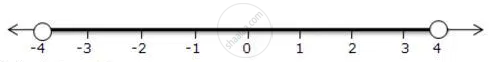Share

# Represent the Following Inequalities on Real Number Lines -4 < X < 4 - Mathematics

Course

#### Question

Represent the following inequalities on real number lines

-4 < x < 4

#### Solution

-4 < x < 4

Solution on number line isIs there an error in this question or solution?

#### APPEARS IN

Selina Solution for Concise Mathematics for Class 10 ICSE (2020 (Latest))
Chapter 4: Linear Inequations (In one variable)
Exercise 4(B) | Q: 1.4 | Page no. 49
Solution Represent the Following Inequalities on Real Number Lines -4 < X < 4 Concept: Linear Inequations in One Variable.
S